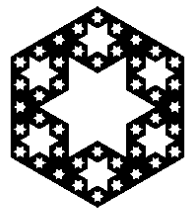# Code to slice open a Menger sponge

Last month the New York Times ran a story about a sculpture based on cutting open a “Menger sponge,” a shape formed by recursively cutting holes through a cube. All the holes are rectangular, but when you cut the sponge open at an angle, you see six-pointed stars.

Here are some better photos, including both a physical model and a computer animation. Thanks to Mike Croucher for the link.

I’ve written some Python code to take slices of a Menger sponge. Here’s a sample output.The Menger sponge starts with a unit cube, i.e. all coordinates are between 0 and 1. At the bottom of the code, you specify a plane by giving a point inside the cube and vector normal to the plane. The picture above is a slice that goes through the center of the cube (0.5, 0.5, 0.5) with a normal vector running from the origin to the opposite corner (1, 1, 1).

```from math import floor, sqrt
from numpy import empty, array
from matplotlib.pylab import imshow, cm, show

def outside_unit_cube(triple):
x, y, z = triple
if x < 0 or y < 0 or z < 0:
return 1
if x > 1 or y > 1 or z > 1:
return 1
return 0

def in_sponge( triple, level ):
"""Determine whether a point lies inside the Menger sponge
after the number of iterations given by 'level.' """
x, y, z = triple
if outside_unit_cube(triple):
return 0
if x == 1 or y == 1 or z == 1:
return 1
for i in range(level):
x *= 3
y *= 3
z *= 3

# A point is removed if two of its coordinates
# lie in middle thirds.
count = 0
if int(floor(x)) % 3 == 1:
count += 1
if int(floor(y)) % 3 == 1:
count += 1
if int(floor(z)) % 3 == 1:
count += 1
if count >= 2:
return 0

return 1

def cross_product(v, w):
v1, v2, v3 = v
w1, w2, w3 = w
return (v2*w3 - v3*w2, v3*w1 - v1*w3, v1*w2 - v2*w1)

def length(v):
"Euclidean length"
x, y, z = v
return sqrt(x*x + y*y + z*z)

def plot_slice(normal, point, level, n):
"""Plot a slice through the Menger sponge by
a plane containing the specified point and having
the specified normal vector. The view is from
the direction normal to the given plane."""

# t is an arbitrary point
# not parallel to the normal direction.
nx, ny, nz = normal
if nx != 0:
t = (0, 1, 1)
elif ny != 0:
t = (1, 0, 1)
else:
t = (1, 1, 0)

# Use cross product to find vector orthogonal to normal
cross = cross_product(normal, t)
v = array(cross) / length(cross)

# Use cross product to find vector orthogonal
# to both v and the normal vector.
cross = cross_product(normal, v)
w = array(cross) / length(cross)

m = empty( (n, n), dtype=int )
h = 1.0 / (n - 1)
k = 2.0*sqrt(3.0)

for x in range(n):
for y in range(n):
pt = point + (h*x - 0.5)*k*v + (h*y - 0.5)*k*w
m[x, y] = 1 - in_sponge(pt, level)
imshow(m, cmap=cm.gray)
show()

# Specify the normal vector of the plane
# cutting through the cube.
normal = (1, 1, 0.5)

# Specify a point on the plane.
point = (0.5, 0.5, 0.5)

level = 3
n = 500
plot_slice(normal, point, level, n)
```

Related post: A chip off the old fractal block

## 4 thoughts on “Code to slice open a Menger sponge”

1. Robert Dickau

Much-belated none-of-my-business, but: Menger sponge slices demonstration, thanks to you.

2. Joshua Zucker

Robert Dickau’s diagrams are a better explanation of why we get the stars than anyone else’s I’ve seen. Thanks for sharing those!